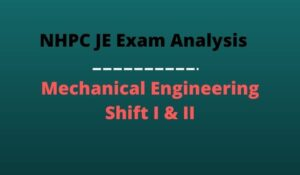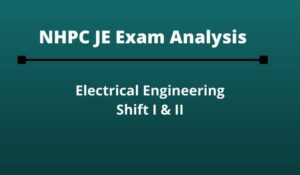Engineering Jobs   »   Civil Engineering quizs   »   NHPC-JE

# NHPC-JE’21 CE: Daily Practices Quiz. 23-Sep-2021

Know your strengths and practice your concepts with this quiz on NHPC JE Recruitment 2021. This quiz for NHPC JE Recruitment 2021 is designed specially according to NHPC Syllabus 2021.

Quiz: Civil Engineering
Exam: NHPC-JE
Topic: Miscellaneous

Each question carries 1 mark
Negative marking: 1/4 mark
Time: 8 Minutes

Q1. The point of contraflexure is the point where:
(a) bending moment changes sign
(b) bending moment is maximum
(c) bending moment is minimum
(d) shear force is zero

Q2. A slab is reinforced with 8 mm ϕ bars and development length required is 548 mm. the support width should be
(a) 275 mm
(b) 183 mm
(c) 208 mm
(d) 548 mm

Q3. The rain is called as acid rain, when its pH is less than_______
(a) 3
(b) 4.5
(c) 7
(d) 8.5

Q4. The road intersection where all converging vehicles are forced to move round a large central island in one direction before they can weave out of traffic flow into their respective directions radiating from the central island is called as __________.
(a) Clover leaf
(b) Staggered intersection
(c) Rotary intersection
(d) Skewed intersection

Q5. A fully saturated soil has a water content of 200 percent. If G = 2.6, the void ratio is:
(a) 1.3
(b) 2.6
(c) 5.2
(d) None of these above

Q6. The magnitude of the normal stresses in the x and y direction is 100 MPa and 20 MPa respectively. both the stresses and tensile in nature. Determine the radius of the Mohr’s circle (mm).
(a) 20
(b) 40
(c) 60
(d) 80

Solutions

S1. Ans.(a)
Sol. Point of contraflexure (POC) is the point where bending moment changes sign.
→ When function changes from concave to convex or vice versa that point is called point of inflection.

S2. Ans.(c)
Sol. the support width = Ld/3+effective cover
=548/3+25
=207.66
≈208 mm.

S3. Ans.(b)
Sol. The rain is called as acid rain, when its pH is less than 4.5. then main cause of acid rain is Sulphur oxides (SOx) and Nitrogen oxides (NOx).

S4. Ans.(c)
Sol. The road intersection when all converging vehicles are forced to move round a large central island in the one direction before they can weave out to traffic flow into their respective directions radiating from the central island is called as rotary intersection.

S5. Ans.(c)
Sol. water content (w) = 200%
G= 2.6
Void Ratio (e) = ?
→ soil is fully saturated (S = 1)
Se = WG
1×e = 2×2.6
▭(e=5.2)

S6. Ans.(b)
Sol. Given that,
σ_x=100 MPa
σ_y=20 MPa
R=√(((σ_x-σ_y)/2)+τ_xy^2 )
R=√(((100-20)/2)^2+0^2 )
R=√((40)^2 )
R=40 mm

Sharing is caring!

### TOPICS:

•NHPC Junior Engineer Syllabus 2023, Chec...
•NHPC JE Exam Pattern 2023, Latest Markin...
•NHPC JE Syllabus 2023, Download Latest S...
•NHPC Result Through GATE 2021, Download ...
•NHPC JE Mechanical Exam Analysis 2022 Di...
•NHPC JE Exam Analysis 2022 Electrical En...